Quick Tools Colection for iOS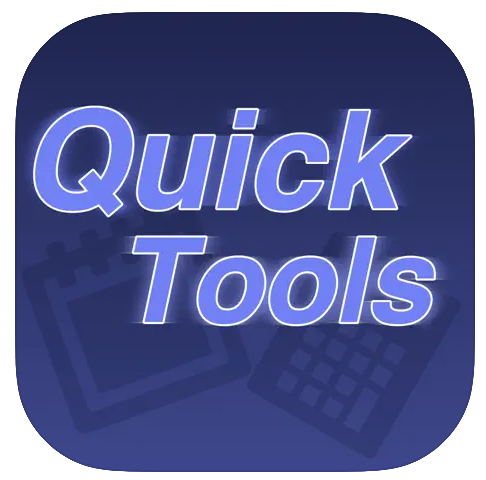Available now on Apple AppStore Apps Privacy Policy Four Quick tools in one apps. Quick Percentage quickest way to get all possible result from percentage calculation. You will get the result of the actual percentage from the amount, the result of the amount minus the amount percentage - like the discounted price and the result of the amount with the amount percentage like the mark-up value. Quick Day fastest and easiest way to get result for date calculation. Useful for calculating loan contracts by days, weeks or even months, or calculate date in week input. Quick Count Simple yet practical quick counting tools. Quick Date The easiest way to find differences between two dates.1. Quick Percentage QuickPercentage Start Screen. A fastest way to get result for Percentage calculation. Just enter the amount and select percentage. Using real-time concept where any changes in any of the input values will automatically re-calculate the result.QuickPercentage sample 1. In the sample below, the amount selected was 750.00 And the Percentage was 15.00% The result will produce all 3 possible usage First is the amount of the percentage, Second is the amount minus the percentage, like Discounted value Lastly is the amount with the percentage, like the mark-up value.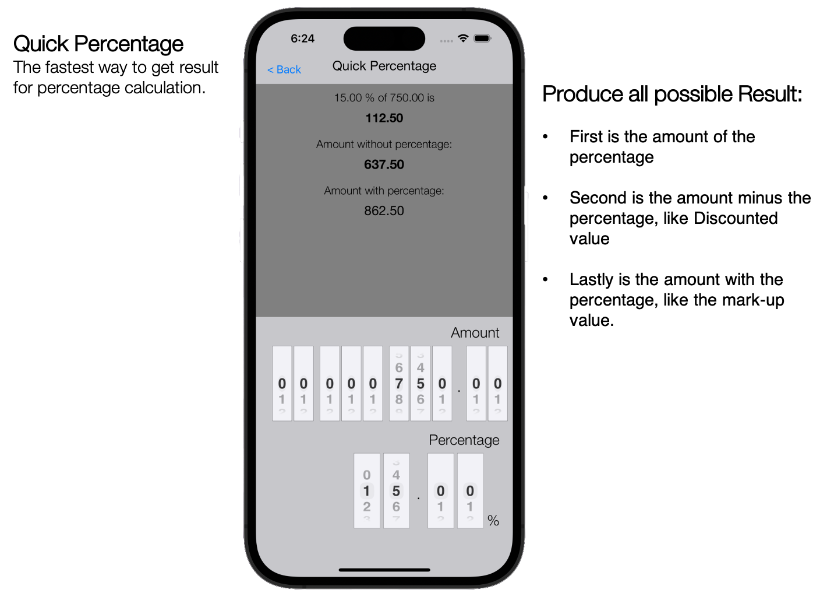QuickPercentage sample 2. Another sample for calculation of 2.5% of 28,000.00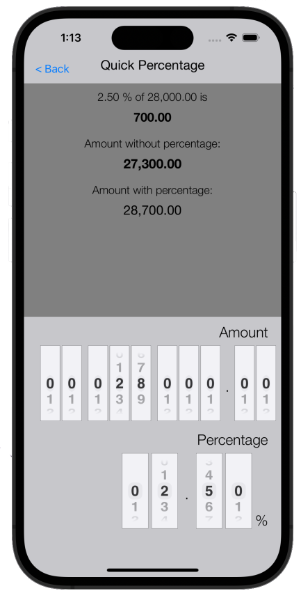2. Quick Day QuickDay Start Screen. Select your preferred date from the Calendar Choose either to calculate Future "+" or Past "-" Choose your numbers and lastly Pick either Month, Week or Days Tap the "Back" button to go back to Main Menu Using real-time concept where any changes in any of the input values will automatically re-calculate the result.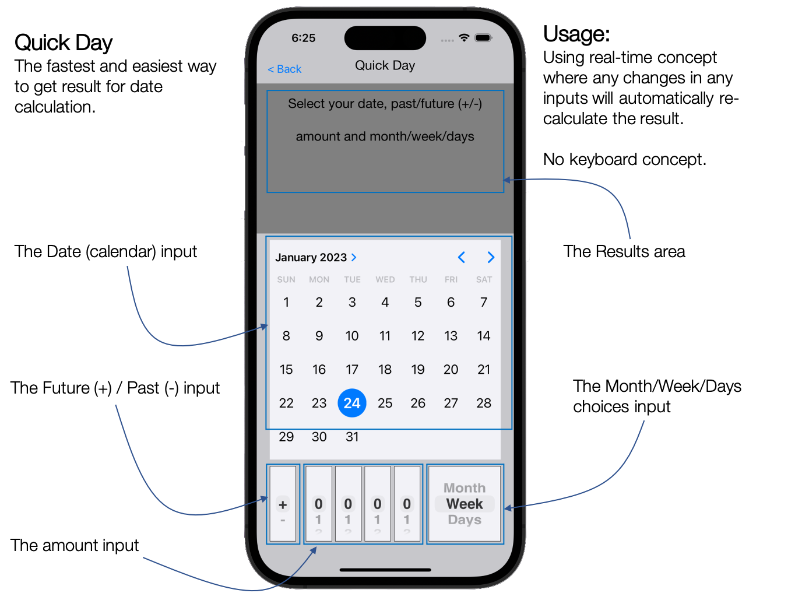QuickDay sample 1. In this sample, the user input the date as 13th-January-2023 and select "8" and "Week", the "+" (future) is default. The result is as shown on the top spaces Changes in any of the input values will automatically re-calculate the result.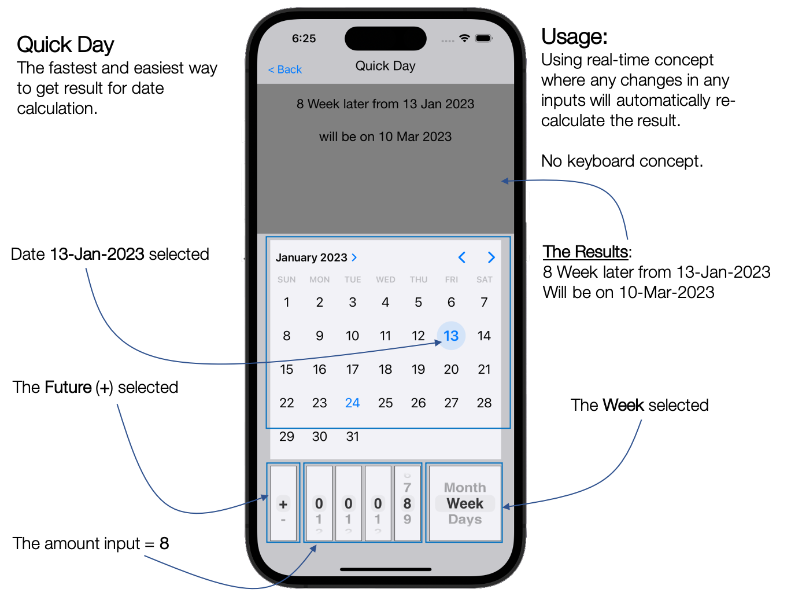QuickDay sample 2. In this sample, the user input the date as 13th-January-2023 and select "8" and "Week", But now the "-" (past) is selected, so the date will be calculated backward. The result is as shown on the top spaces Changes in any of the input values will automatically re-calculate the result.QuickDay sample 3. In this sample, the user input the date as 16th-January-2023 and select "132" and "Days",3. Quick Count QuickCount Start Screen. Press "Back" button to go back to main menu Tap the center big button to start the counting Tap the "minus" button to back-track the counting Tap the "Reset" button to reset the counting back to zeroQuickCount sample 1. Tapping the counter box will increase the counting,and tapping the "minus" button will backtrack the counting,QuickCount reset counting. Pressing the "Reset" button will prompt the user a confirmationto reset the current counting.Press "Reset" to confirm reset to zero orpress "Cancel" to back to current counting.4. Quick Date QuickDate sample 1. In this sample, the user input the first date as 21th-February-2023 and select the second date as 05-December-2024, The result is as shown on the top spaces Changes in any of the input values will automatically re-calculate the result.© 2023 StarAhead.com. All rights reserved.